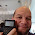## Sunday, November 18, 2012

### Graphing Calculator Programming Languages Comparison: TI 84 Plus vs Casio Prizm

This is a short comparison between the programming languages of the Texas Instruments TI-84+ and the Casio Prizm. For each category, I will list the different syntaxes required.

If you find a program that you like that is programmed on a calculator you don't have, no fear! This guide can be served as a translation guide. For more details, consult the manual or search for detailed tutorials.

Hope this helps,

Eddie

Notes:

The commands for the TI-84+ also covers the TI-84+ Silver Edition, TI-83+ Silver Edition, TI-83+, and most likely the TI-82 and the upcoming TI-84+ Plus C Silver Edition (84+ with a color screen). The TI-84+ family carries a program memory of about 24,000 bytes, 28,000 for the TI-82.

The commands for the Casio Prizm (Model fx-CG 10/20) also apply to the fx-9860g (all versions), fx-9750g, and (most likely) fx-9850g. The Casio family carriers about 60,000-62,000 bytes of program memory, except the 9850g (32,000 bytes).

Keystrokes may vary.

Arguments in each command are in italics.

Here we go:

Numerical Derivative

TI-84+: MATH, 8
nDeriv(f(variable),var,value)

Casio Prizm: OPTN, F4, F2
d/dx(f(X),value)
The variable is always X.

Definite Integral

TI-84+: MATH, 9
fnInt(f(var), var, lower limit, upper limit)

Casio Prizm: OPTN, F4, F4
∫(f(X),lower limit,upper limit)
The variable is always X.

Solve

TI-84+: Catalog or MATH, B in programming mode
solve(expression,variable to be solved for,guess, range*)
expression is set to be equal to 0
range is a two element list {low, high}, and is an optional argument.

Casio Prizm:

There are two commands.

Solve f(X)=0 for X: OPTN, F4, F1
Solve(f(X),guess,low,high)

Solve an equation in any variable: OPTN, F4, F5
SolveN(equation,variable to be solved for,low,high)

Sums

TI-84+: MATH, 0
Σ(f(var),var,start value,end value)

Casio Prizm: OPTN, F4, F6, F3
Σ(f(var),var,start value,end value)

TI-84+:
Input "prompt string", var

Casio Prizm:
"prompt string"? → var

Displaying Results

TI-84+:
Disp var or string
You can add other lines, using commas to separate them.

Pause var
Pause allows the user to scroll the variable's value.

Casio Prizm:
var or string
The ◢ is the right triangle symbol, which acts like a pause command.

If Then Else

TI-84+:
If test expression
Then
do this if test is true
Else
do this if test is false
End

Casio Prizm:
If test expression
Then
do this if test is true
Else
do this if test is false
EndIf

Shortcut: Jump Command
test condition1 command if test is true : skip to here if the test is false

For Loop

TI-84+:
For(counter var,start value,end value,step size*)
commands
End

step size can be positive or negative, and is optional

Casio Prizm:
For start valuevar To end value Step step size*
commands
End

step size can be positive or negative, and is optional

While Loop

TI-84+:
While this test condition is true
do these commands
End

Casio Prizm:
While this test condition is true
do these commands
WhileEnd

Do Until Loop

TI-84+:
Repeat until this condition becomes true
these commands
End

Casio Prizm:
Do
these commands
LpWhile this condition remains false

List and Matrix Element Calls

TI-84+:
Lists:
L#(element number)
#: 1 through 6 or custom name

Matrix:
[[#]](row,column)
#: A through J

Casio Prizm:
Lists:
List #[element number]
#: 1 through 26 or custom name

Matrix:
Mat #[row,column]
#: 1 through 26

Giving the user a menu of options

TI-84+:

Casio Prizm:
Menu "title string", "choice 1", label name...

Decrementing and Incrementing Variables by 1

TI-84+:
IS>(var,target value)
do if var + 1 ≤ target value

DS<(var,target value)
do if var - 1 ≥ target value

Casio Prizm:
ISZ var
do if var + 1 ≠ 0

DSZ var
do if var - 1 ≠ 0

This blog is property of Edward Shore, 2012.

1.Hi there! Thanks for the informative blog. I would like to confirm whether Casio PRIZM allow integration with limits? and integration by parts?

2.Thank you Wellwisher. The PRIZM only works with numerical calculations.

3.It’s not easy to find such perfectly written information on this topic. Great Work,check out for modern calculator @ http://www.moderncalculator.com

### Σ(1 / (a^n)) from n=1 to m

Σ(1 / (a^n)) from n=1 to m This blog entry covers the sum of the series: Σ[1 / (a^n), n=1 to m] with n and m positive integers Specific Cas...